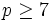# Abelian-to-normal replacement fails for half of prime plus nine for prime greater than five

This article discusses a failure of replacement, i.e., a situation where the analogue of a valid replacement theorem fails to hold under slightly modified conditions.
View other failures of replacement | View replacement theorems

## History

This result is part of an as yet unpublished paper by George Glauberman.

## Statement

Suppose$p$ is a prime number greater than$5$ (in other words,$p \ge 7$). Suppose$k \ge (p + 9)/2$. Then, there exists a finite$p$-group$P$ having an abelian subgroup of order$p^k$ but no abelian normal subgroup of order$p^k$.